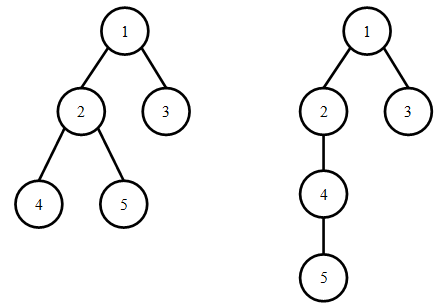## Day 1, Problem 2 - Tree Count

We know that a rooted tree can be traversed via depth-first search (DFS) and breadth-first search (BFS) to obtain the DFS and BFS orderings of their vertices. Two different trees can have the same DFS ordering, and at the same time their BFS orderings can also be the same. For example, the following two trees both have a DFS ordering of 1 2 4 5 3 and a BFS ordering of 1 2 3 4 5.Given a DFS and BFS ordering, we would like to know the average height of all rooted trees satisfying the condition. For example, if there are K different rooted trees simultaneously possessing the DFS and BFS orderings, and their heights are respectively h1, h2, …, hK, then you are asked to output the value of (h1 + h2 + … + hK)/K.

### Input Format

The first line contains a single positive integer n, representing the number of vertices.
The second line contains n positive integers (each between 1 and n, inclusive), representing the DFS ordering.
The third line contains n positive integers (each between 1 and n, inclusive), representing the BFS ordering.
The input guarantees that at least one tree satisfying the two orderings will exist.

### Output Format

Output a single real number, rounded half-up to three places after the decimal point, representing the average height of the trees.

```5
1 2 4 5 3
1 2 3 4 5
```

### Sample Output

```3.500
```

If your output differs from the correct answer by no more than 0.001, then you will receive full marks on the test case. Otherwise you will receive no marks.

### Constraints

20% of the test cases will satisfy n ≤ 10;
40% of the test cases will satisfy n ≤ 100;
85% of the test cases will satisfy n ≤ 2000;
100% of the test cases will satisfy 2 ≤ n ≤ 200000.

### Hints

If a rooted tree has only one vertex, then its height is 1. Otherwise, it's height is equal to 1 plus the maximum height across all of the subtrees rooted at each children.

For any three vertices a, b, and c in the tree, if a and b are both c's children, then the relative orders of a and b in both the BFS and DFS orderings are the same. That is, either a is always before b, or a is always after b.

Point Value: 25 (partial)
Time Limit: 1.00s
Memory Limit: 256M

Languages Allowed:
C++03, PAS, C, HASK, ASM, RUBY, PYTH2, JAVA, PHP, SCM, CAML, PERL, C#, C++11, PYTH3